HOME / Applications / 3D FEM simulation of a DC non-linear Contactor

# 3D FEM simulation of a DC non-linear Contactor

Used Tools:## Introduction

Magnetic actuators are based on electromagnetic field to convert electrical energy into mechanical energy. Depending on the type of generated motion; linear or rotational movement, electromagnetic actuators are classified in two categories: rotary and linear actuator.
Among the applications of DC actuators, we find the electromagnetic contactors based on linear motion. They are widely used for making and breaking connections as switchgear equipment for electrical power sources.  They are generally composed of permanents magnets, solenoids and ferromagnetic parts.

In many industrial applications, the energy-saving performance of devices is often a very attractive benefit. Particularly, a new type of DC contactor electronically controlled, associated with a permanent magnet has drawn more and more attention in recent years because of its outstanding benefits, such as energy-saving and noise-free characteristics.

The studied DC contactor is made of three parts: a non-linear ferromagnetic core, with its two moving and fixed parts, excited by a DC copper coil, like demonstrated in the figure 1.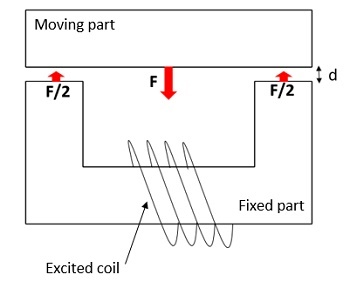Figure 1 - Schematic illustration of the DC contactor

## Problem description

The Magnetostatic module of EMS is employed to compute the generated force across the air gap between the lower and upper moving part of the nonlinear ferromagnetic core. The simulated model has a translational invariance along the z axis. The work coil is made of 1000 turns of copper wires carrying a DC current of 2A. The air gap between the fixed and moving part is set to 5 mm. The 3D design of the studied model with the detailed dimensions are shown in the figure2.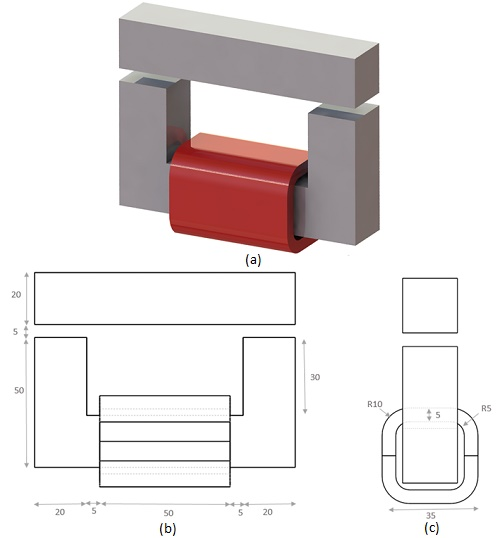Figure 2 - a) 3D design, b) frontal and c) side view of the DC contactor

The physical properties of the copper used for the work coil, are defined in the table 1 below:
Table 1 - Material properties
 Material Density () Magnetic permeability Electrical conductivity (S/m) Thermal conductivity (W/m.K) Specific heat capacity (J/Kg.K) Copper (Cu) 8900 0.99 6 E+07 385 390

The core is made of nonlinear ferromagnetic material, for which the BH relation can be defined by the expression below:With:: Saturation value of B field: Initial permeability: Vacuum permeability

The corresponding BH curve is defined by the next 2D plot: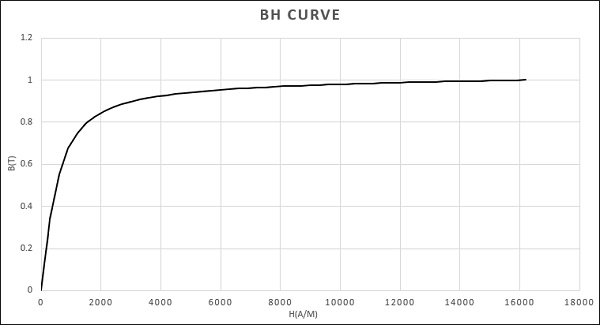Figure 3 - BH curve

Mesh quality is critical to every FEM simulation. The results accuracy and the solving time are strongly dependent on the mesh size. EMS allows user to control the mesh size on solid bodies and faces through its Mesh control feature. In this example, a fine mesh control
Was applied to our studied model for more precise results.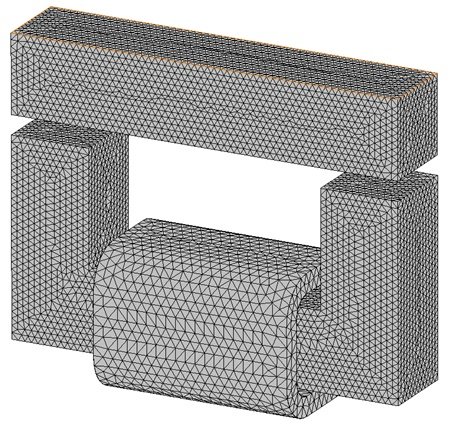Figure 4 - The meshed model

## Results

The work principle of the magnetic contactor consists of passing a DC current through a multi turn coil which can generate a static magnetic flux. The magnetic flux density will produce a constant magnetic force on the moving part of the ferromagnetic core. The Magnetostatic simulation revealed the results shown in the figures below.

### 1.Electromagnetic analysis

The distribution of flux density across the ferromagnetic core for a current excitation of 2A achieves a maximum value of 0.98T like shown in the figure 5.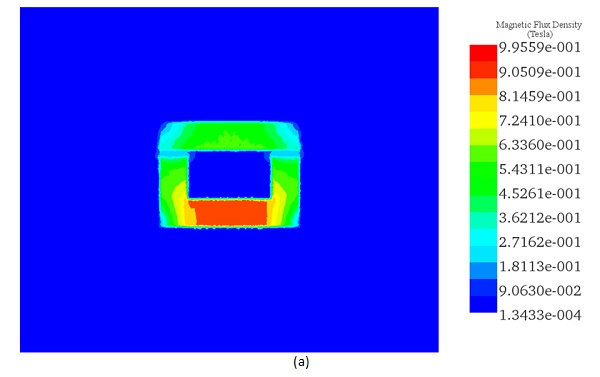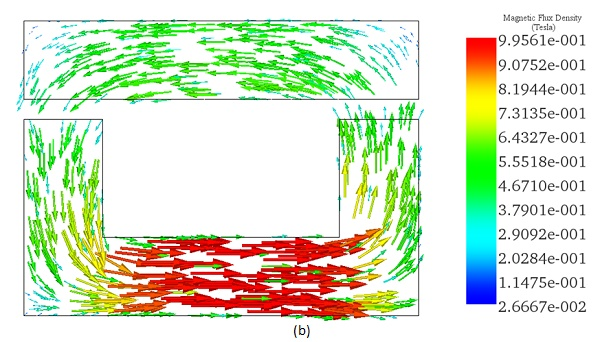Figure 5 - a) Fringe and b) vector plot of Magnetic flux distribution

The magnetic force distribution across the area between the moving and fixed part is shown by the figure 6. It is computed inside EMS through virtual work feature.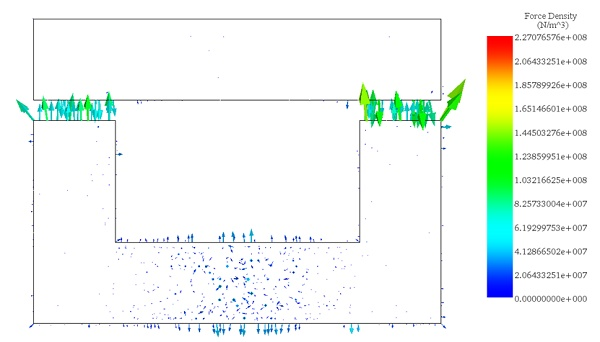Figure 6 - vector plot of force density distribution

### 2.Electromagnetic analysis coupled to SOLIDWORKS motion

By coupling the Magnetostatic module of EMS with motion inside SOLIDWORKS, the solver of SW Motion uses the computed force and converts it into a mechanical motion. It allows to visualize the variation of the moving part position versus time.
Under a fixed current excitation of 2A, and after t=3.5 ms, the simulation revealed the next results. The variation of the generated force and the Center of mass position of the moving part versus time are given by figure 7. This position is giving an idea about the air gap variation between the two parts of the magnetic contactor versus time. It is decreasing from d=5mm to d=2.3 mm.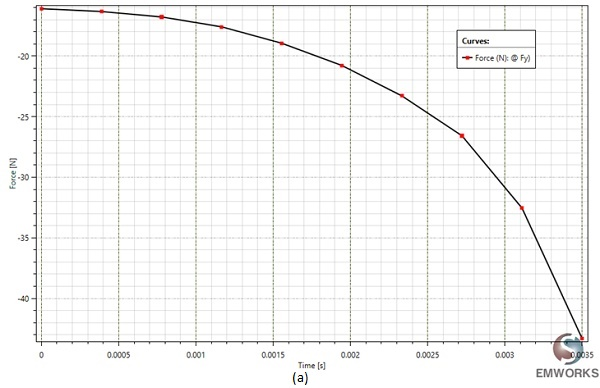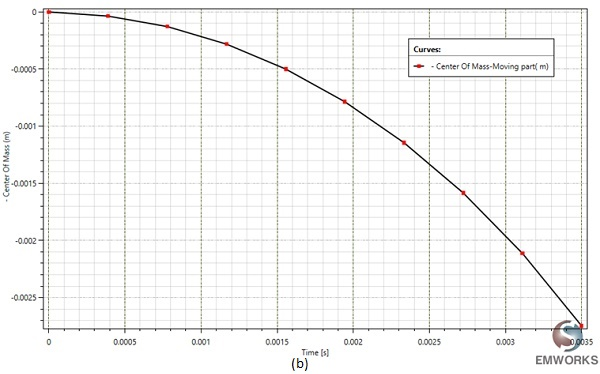Figure 7 - a) Force variation and b) Center of mass position of the moving part versus time.

The instantaneous linear velocity could be computed by SOLIDWORKS motion analysis and visualized by EMS results table. It has a maximum value of 1.77 m/s at t=3,5ms. It acts proportionally to the generated force.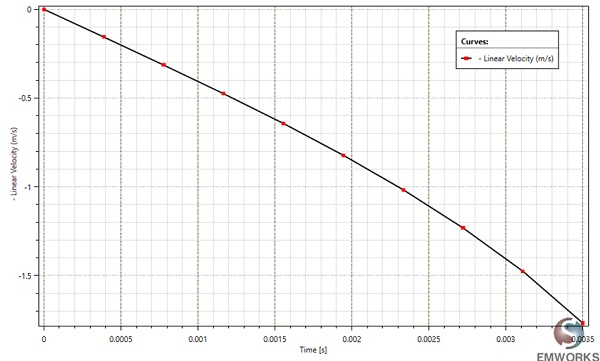Figure 8 - Linear velocity variation versus time.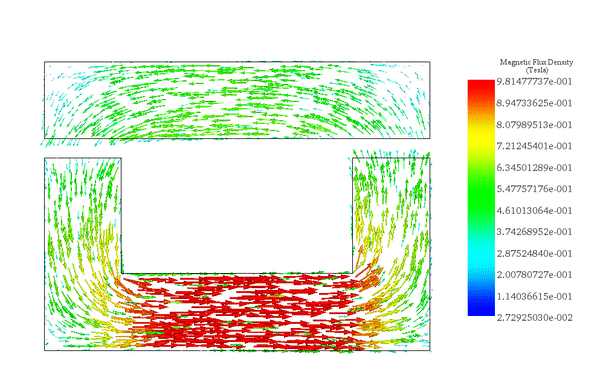Figure 9 - Magnetic flux density animation versus the moving part displacement

### 3.Parametric analysis

EMS allows to analyze the several aspects of the studied electromagnet through the run of different scenarios in a single study by parameterizing both simulation and geometrical variables.

This feature is used to compute the generated force of the studied contactor versus current excitation and air gap distance between the two parts.

The selected parametric variables are shown in the next table:

Table 2 - Simulation scenarios
 Scenarios Air gap length (mm) Current (A) scenario 1 1 1 scenario 2 2 1 scenario 3 3 1 scenario 4 4 1 scenario 5 1 2 scenario 6 2 2 scenario 7 3 2 scenario 8 4 2 scenario 9 1 3 scenario 10 2 3 scenario 11 3 3 scenario 12 4 3 scenario 13 1 4 scenario 14 2 4 scenario 15 3 4 scenario 16 4 4

By varying both current and air gap distance, EMS computes and generates the magnetic force in the upper moving part of the contactor. Figure 10 shows 3D plot of the force results. The resultant force increases with higher current and smaller gap distance.

The studied magnetic actuator is showing significant amounts of magnetic forces under low power consumption, which makes this category of switchers more popular compared to other conventional types.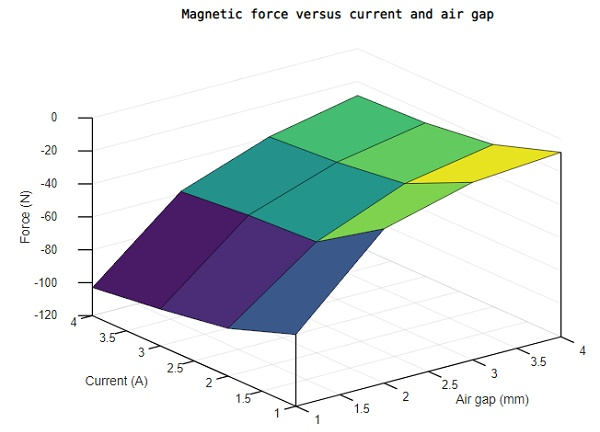Figure 10 - 3D graph of the generated force versus Current and Air gap.

## Conclusion

A non-linear magnetostatic problem was investigated using EMS simulation software. The magnetic force over a DC contactor, was computed versus many current excitations and for different air gap distances.

To optimize the design and the efficiency of such Electromagnetic actuators, evaluating the generated magnetic forces is highly required to achieve more accurate results for several scenarios of the switchgear equipment. As well, With the increasing concern on reliability and life time of electromagnetic contactors, manufacturers are now working on new devices equipped with electronic control to avoid problems related to contact bounce, excessive heating and even contact welding . It allows to bring both performance and reliability to the conventional electromagnetic contactors.

## References

. Le Van, V., 2015. Développement de formulations intégrales de volume en magnétostatique (Doctoral dissertation, Université Grenoble Alpes).
. Perin, Arnaldo JosÉ. "An electronic control unit for reducing contact bounce in electromagnetic contactors." IEEE Transactions on Industrial Electronics 55.2 (2008): 861-870.

Upcoming Webinars